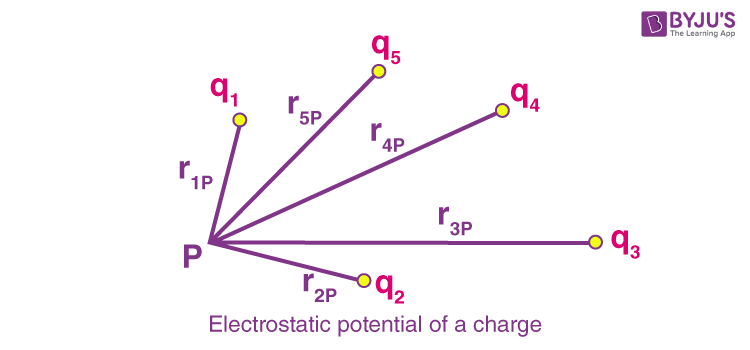# Electrostatic Potential

The effort required to move a unit positive charge (without acceleration) from infinity to any point in an electrostatic field is represented by the electrostatic potential (V) at that location. In this article, we will be studying electrostatic potential.

## What Is Electrostatic Potential?

The electrostatic potential, also known as the electric field potential, electric potential, or potential drop is defined as

The amount of work done to move a unit charge from a reference point to a specific point inside the field without producing an acceleration.

### SI Unit of Electrostatic Potential

The SI unit of electrostatic potential is volt.

 SI unit of electrostatic potential volt Other units statvolt Symbol of electrostatic potential V or φ Dimensional formula ML2T3I-1

## Electric Potential Formula

The following formula gives the electric potential energy of the system:

 $$\begin{array}{l}U=\frac{1}{4\pi \varepsilon _{0}}\times \frac{q_{1}q_{2}}{d}\end{array}$$

Where q1 and q2 are the two charges that are separated by the distance d.

## Electrostatic Potential of A Charge

Let us consider a system of charges consisting of static charges, that is, charges fixed at a position that does not undergo any type of motion. In this system, we define the potential energy of a charge in terms of the work done on the charge. As we know, when a charge, say q, is placed in an electric field E (the electric field at any point due to the charge configuration of the system), it experiences a force proportional to the magnitude of its charge equal to q×E. If we divide the resultant work done by the magnitude of charge, it becomes independent of the charge. This is how we calculate the electrostatic potential of a charge. In this section, we will learn about the electrostatic potential of a charge.Taking an example of a charged particle being brought from a point A to a point B in the electric field, the work done by an external force in bringing this unit positive charge from point A to B is given by,

$$\begin{array}{l} V _ { B } \, – \, V _ { A } = \frac{ U _ { B } \, – \, U _ { A } }{ q } \end{array}$$

Where VA and VB is the electrostatic potential of the particle at points A and B, respectively, UA and UB are the potential energy of the particle at points A and B. Q is the magnitude of the charge.

As we know, the actual value of the potential at any point holds no significance, and we would rather calculate the potential difference between two points for any given system of charges.

Now, as we know, the potential energy of a charged particle at infinity is assumed to be zero, so the electrostatic potential of an object is calculated for a particle with infinity reference.

Electrostatic potential in the electric field region, at any point, is defined as the work done in bringing a unit charge from infinity to that point such that the particle undergoes no acceleration.

## See the video below to learn important JEE questions on electrostatic potential and capacitance## Frequently Asked Questions – FAQs

Q1

### What is electrostatic potential also known as?

The term “potential drop,” “electric field potential,” and “electric potential” are all names for the electrostatic potential.
Q2

### What is the SI unit of electrostatic potential?

The volt is the SI unit of electrostatic potential.
Q3

### What is the dimensional formula of electrostatic potential?.

Dimensional formula of electrostatic potential is ML2T3I-1.
Q4

### What are the symbols used to represent the electrostatic potential?

V or φ are the symbols used to represent the electrostatic potential.
Q5

### What is the formula to calculate the electric potential energy?

The formula gives the electric potential energy:
$$\begin{array}{l}U=\frac{1}{4\pi \varepsilon _{0}}\times \frac{q_{1}q_{2}}{d}\end{array}$$

## Watch the video and solve important questions in the chapter Electricity Class 10